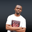RELATED TAGS

euphoria

# What is the series() method in Euphoria?NDUKWE CHIDERA K.

## Overview

Building a series is a common arithmetic operation where a sequentially ordered set of numbers is produced following a laid-down pattern. We can build a simple geometric or linear series in Euphoria using the series() method.

The series() method is used to generate a geometric or a linear series. It does so by using a start_value that is the initial member of the series. Then, it increments it with the increment until a certain amount of series items are generated. This amount is determined by count. The operator parameter indicates the type of series (linear or geometric).

### Syntax

series(start,increment,count,operator)


### Parameters

• start: This is an object and is the first value of the series to be returned. It is a required parameter.
• increment: This is also an object and a required parameter. It is the value by which the series will be incremented either linearly or geometrically. A linear increment is done by adding the value to the last element of the series to get the next one. A geometric increment is done by multiplying the value with the last element of the series at any point in time to get the next.
• count: This is an integer value and a required parameter. It indicates the number of items generated by the series.
• operator: This is the arithmetic operator which acts as the series builder. It is an integer value. This parameter is optional and has a default value of +.

### Return value

This function will return a new sequence series built from the object supplied.

Note: When a negative value is supplied as a count or the increment value provided is not a valid object, 0 is returned. However, with a valid count, a sequence is returned whose length is equal to the count. This is the length of the returned count sequence.

### Example

--include some modules needed
include std/sequence.e

--define some variables
object start = 2
object increment = 3
integer count = 10
integer operator = 42

--save the output of the series method in some variables
sequence output1 = series(start,increment,count)
sequence output2 = series(start,increment,count,operator)

--display the outcome of using the series.
print(1,output1)
puts(1,"\n")
print(1,output2)
An example of the series() method

### Explanation

The second series is a geometric series formed using the multiplication operator indicated by its integer value 42. This integer value is obtained by printing print(1,"*"). It gives an integer representation of any operator in Euphoria.

• Line 2: We include the sequence.e module from the standard library.
• Lines 5–8: We define the necessary variable.
• Lines 11 and 12: We use the series() method to create a series.
• Lines 15–17: We display the output.

RELATED TAGS

euphoria

CONTRIBUTORNDUKWE CHIDERA K.
RELATED COURSES

View all Courses

Keep Exploring

Learn in-demand tech skills in half the time